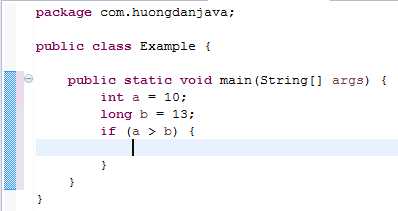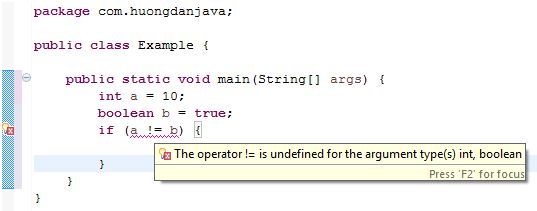# Relational operators in Java

Relational operators in Java are used to test whether a condition is satisfied. They consist of the following six operators:

• >
• >=
• <
• <=
• ==
• !=

### >, >=, <, <= operators

We use the operators >, >=, <, <= to check that this primitive value is greater than or equal to or less than the other primitive value. These operators can be used for all primitive data types except the boolean data type.

For example:

The result of the above example is false because 129 is less than 312.

We cannot use relational operators to compare two incompatible primitives. Incompatibility means that one of two values cannot be automatically converted to the other.

For example:In the above example, int a variable can be automatically converted to long so we can use the relative operator for comparison.

For the example below, the relational operator cannot be used:Because boolean or int types cannot be converted between them.

### ==, != operators

The operators == or != can be used for all primitive data types.

The == operator returns true if two values are equal and the opposite is false. In contrast to the == operator, the != statement returns true if the two values are not equal, and false is equal.

For example:

The result will be false because 12 is not equal to 13.

We also cannot use the ==, != operators for incompatible primitives.

For example: